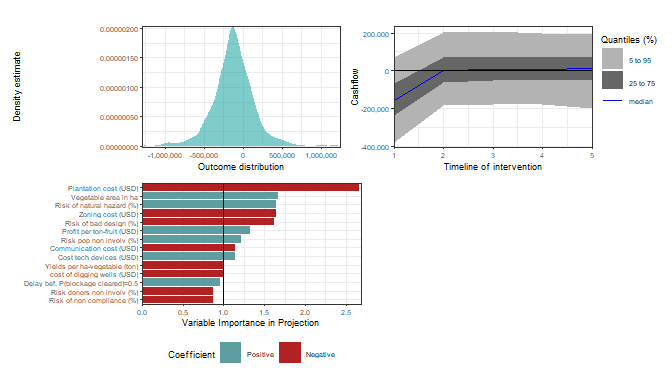# Applying the mcSimulation function in decisionSupport

### Decision analysis with the decisionSupport package

#### The decisionSupport package

Many of the variables decision makers need to consider in development cannot be precisely quantified, at least not without unreasonable effort. The major objective of (prescriptive) decision analysis is to support the decision-making processes when faced with this problem (E. Luedeling and Shepherd 2016). Decision analysis can make forecasts of decision outcomes without precise numbers.

The decisionSupport package (Eike Luedeling et al. 2022) implements this as a Monte Carlo simulation, which generates a large number of plausible system outcomes, based on random numbers for each input variable, drawn from user-specified probability distributions.

1. an R function that predicts decision outcomes based on the variables named in a separate data table. This R function is customized by the user to address a particular decision problem to provide the decision analysis model.
2. an input table (in .csv format) specifying the names and probability distributions for all variables used in the decision model. These distributions aim to represent the full range of possible values for each component of the model.

These two inputs are provided as arguments to the mcSimulation function, which conducts a Monte Carlo analysis with repeated model runs based on probability distributions for all uncertain variables. The data table and model are customized to fit the particulars of a specific decision.

decisionSupport package can be installed from CRAN with the install.packages() function.

install.packages("decisionSupport")

The package can be loaded with the library() function for further use in this example.

library(decisionSupport)

#### Example – Reservoir Sedimentation in the Upper Volta River Basin of Burkina Faso

We showcase a partial example of a Monte-Carlo-based selection of a sedimentation management strategy for a reservoir in the Upper Volta River Basin of Burkina Faso (Lanzanova et al. 2019). These reservoirs have multiple benefits for rural communities and are important for food security and livelihoods. Sedimentation is a major impediment for the functioning of these reservoirs. The design of an efficient sedimentation management intervention requires assessment of multiple uncertain quantities and risks. Decision analysis is highly applicable in such data-scarce environments, where science has traditionally struggled to provide actionable information to policy-makers, development practitioners, NGOs and rural communities.

Here we walk through a simple example of the use of various decisionSupport functions, which use the tidyverse libraries (Wickham et al. 2019) including ggplot2 (Wickham, Chang, et al. 2021), plyr (Wickham 2020) and dplyr (Wickham, François, et al. 2021) among others in the R programming language (R Core Team 2021).

Here we generate a model as a function using decisionSupport library. The example is a simple version of the model applied in Burkina Faso (Lanzanova et al. 2019). We use the decisionSupport functions vv() to produce time series with variation from a pre-defined mean and coefficient of variation, chance_event() to simulate whether events occur and discount() to discount values along a time series.

example_decision_function <- function(x, varnames){

# calculate ex-ante risks: impact the implementation of interventions ####
intervention_NonPopInvolvEvent <-
chance_event(intervention_NonPopInvolv, 1, 0, n = 1)

# pre-calculation of common random draws for all intervention model runs ####

# profits from Tropical Livestock Units (TLU)
TLU <- vv(TLU_no_intervention, var_CV, n_years)
TLU_profit <- vv(profit_per_TLU, var_CV, n_years)

# benefits of fruit
precalc_intervention_fruit_benefits <-
vv(intervention_fruit_area_ha, var_CV, n_years) *
vv(intervention_fruit_yield_t_ha, var_CV, n_years) *
vv(intervention_fruit_profit_USD_t, var_CV, n_years)

# benefits of vegetables
precalc_intervention_vegetable_benefits <-
vv(intervention_vegetable_area_ha, var_CV, n_years) *
vv(intervention_vegetable_yield_t_ha, var_CV, n_years) *
vv(intervention_vegetable_profit_USD_t, var_CV, n_years)

# benefits of rainfed crops
precalc_intervention_rainfed_crop_benefits <-
vv(intervention_rainfed_crop_area_ha, var_CV, n_years) *
vv(intervention_rainfed_crop_yield_t_ha, var_CV, n_years) *
vv(intervention_rainfed_crop_profit_USD_t, var_CV, n_years)

#  Intervention ####

for (decision_intervention_strips in c(FALSE,TRUE))
{

if (decision_intervention_strips)
{
intervention_strips <- TRUE
intervention_strips_PlanningCost <- TRUE
intervention_strips_cost <- TRUE
} else
{
intervention_strips <- FALSE
intervention_strips_PlanningCost <- FALSE
intervention_strips_cost <- FALSE
}

if (intervention_NonPopInvolvEvent) {
intervention_strips <- FALSE
intervention_strips_cost <- FALSE
}

# Costs ####
if (intervention_strips_cost) {
cost_intervention_strips <-
intervention_adaptation_cost + intervention_tech_devices_cost + intervention_nursery_cost +
intervention_wells_cost +
intervention_training_cost + intervention_mngmt_oprt_cost + intervention_mngmt_follow_cost +
intervention_mngmt_audit_cost
} else
cost_intervention_strips <- 0

if (intervention_strips_PlanningCost) {
plan_cost_intervention_strips <-
intervention_communication_cost + intervention_zoning_cost
} else
plan_cost_intervention_strips <- 0

maintenance_cost <- rep(0, n_years)

if (intervention_strips)
maintenance_cost <-
maintenance_cost + vv(maintenance_intervention_strips, var_CV, n_years)

intervention_cost <- maintenance_cost
intervention_cost <-
intervention_cost + cost_intervention_strips + plan_cost_intervention_strips

# Benefits from  cultivation in the intervention strips ####

intervention_fruit_benefits <-
as.numeric(intervention_strips) * precalc_intervention_fruit_benefits
intervention_vegetable_benefits <-
as.numeric(intervention_strips) * precalc_intervention_vegetable_benefits
intervention_rainfed_crop_benefits <-
as.numeric(intervention_strips) * precalc_intervention_rainfed_crop_benefits

# Total benefits from crop production (agricultural development and riparian zone) ####
crop_production <-
intervention_fruit_benefits +
intervention_vegetable_benefits +
intervention_rainfed_crop_benefits

# Benefits from livestock ####
# The following allows considering that intervention strips may
# restrict access to the reservoir for livestock.

if (intervention_strips)
TLU_intervention <-
TLU * (1 + change_TLU_intervention_perc / 100)
else
TLU_intervention <- TLU

if (decision_intervention_strips){
livestock_benefits <- TLU_intervention * TLU_profit
total_benefits <- crop_production + livestock_benefits
net_benefits <- total_benefits - intervention_cost
result_interv <- net_benefits}

if (!decision_intervention_strips){
livestock_benefits <- TLU_no_intervention * TLU_profit
total_benefits <- livestock_benefits
net_benefits <- total_benefits - intervention_cost
result_n_interv <- net_benefits}

} #close intervention loop bracket

NPV_interv <-
discount(result_interv, discount_rate, calculate_NPV = TRUE)

NPV_n_interv <-
discount(result_n_interv, discount_rate, calculate_NPV = TRUE)

# Beware, if you do not name your outputs (left-hand side of the equal sign) in the return section,
# the variables will be called output_1, _2, etc.

return(list(Interv_NPV = NPV_interv,
NO_Interv_NPV = NPV_n_interv,
NPV_decision_do = NPV_interv - NPV_n_interv,
Cashflow_decision_do = result_interv - result_n_interv))
}

#### Perform a Monte Carlo simulation

Using the model function above, we can perform a Monte Carlo simulation with the mcSimulation() function from decisionSupport. This function generates distributions of all variables in the input table as well as the specified model outputs (see return() function above) by calculating random draws in our defined example_decision_function(). Make sure that all the variables in the input table are included in the model (erroneous variables listed there can cause issues with some of the post-hoc analyses).

The numberOfModelRuns argument is an integer indicating the number of model runs for the Monte Carlo simulation. Unless the model function is very complex, 10,000 runs is a reasonable choice (for complex models, 10,000 model runs can take a while, so especially when the model is still under development, it often makes sense to use a lower number).

mcSimulation_results <- decisionSupport::mcSimulation(
model_function = example_decision_function,
numberOfModelRuns = 1e3, #run 1,000 times
functionSyntax = "plainNames"
)

#### Plot Net Present Value (NPV) distributions

We can use the plot_distributions() function to produce one of the several plotting options for distribution outputs. This shows us an overlay of the full results of the Monte Carlo model of the decision options, i.e. the expected NPV if we choose to do the intervention Interv_NPV or not do the intervention NO_Interv_NPV.

decisionSupport::plot_distributions(mcSimulation_object = mcSimulation_results,
vars = c("Interv_NPV", "NO_Interv_NPV"),
method = 'smooth_simple_overlay',
base_size = 7)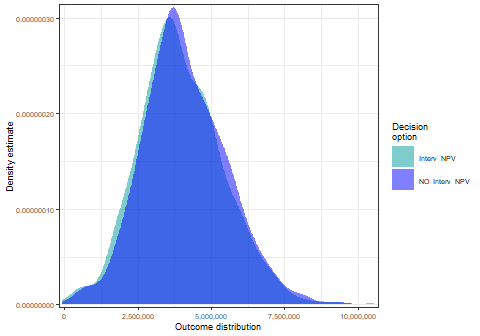We use the same function to show the distributions of the ‘do’ and ‘do not do’ decision scenarios as boxplots. This can be useful when comparing multiple outputs by illustrating the spread of the data resulting from the decision model. Boxplots show the median (central line), the 25th and 75th percentiles (sides of boxes) and any outliers (light circles outside of boxes).

decisionSupport::plot_distributions(mcSimulation_object = mcSimulation_results,
vars = c("Interv_NPV",
"NO_Interv_NPV"),
method = 'boxplot')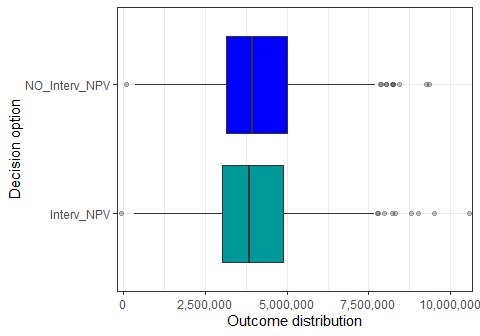We can use the same function for the value of the decision (difference in NPV between do and do not do). This is more helpful for us since it shows us the outcome distribution of the decision itself.

decisionSupport::plot_distributions(mcSimulation_object = mcSimulation_results,
vars = "NPV_decision_do",
method = 'boxplot_density')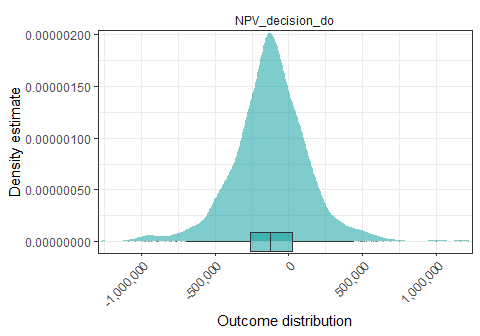#### Cashflow analysis

Here we plot the distribution of annual cashflow over the entire simulated period for the intervention. For this we use the plot_cashflow() function which uses the specified cashflow outputs from the mcSimulation() function (in our case Cashflow_decision_do) to show cashflow over time.

plot_cashflow(mcSimulation_object = mcSimulation_results, cashflow_var_name = "Cashflow_decision_do")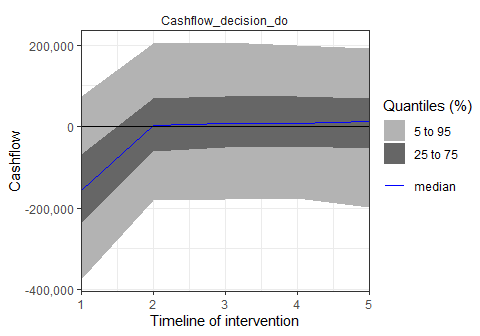#### Projection to Latent Structures (PLS) analysis

We apply a post-hoc analysis to the mcSimulation() outputs with plsr.mcSimulation() to determine the Variable Importance in the Projection (VIP) score and coefficients of a Projection to Latent Structures (PLS) regression model. This function uses the outputs of the mcSimulation() selecting all the input variables from the decision analysis function in the parameter object and then runs a PLS regression with an outcome variable defined in the parameter resultName. We use the code names(mcSimulation_results$y) to select the outcome variable NPV_decision_do, which is the third element of the list y in our mcSimulation_results outputs (this must be a character element). pls_result <- plsr.mcSimulation(object = mcSimulation_results, resultName = names(mcSimulation_results$y), ncomp = 1)

We run the plot_pls() on the results from plsr.mcSimulation() with a number of standard settings. The length of the bars is equal to VIP with a vertical line at ‘1’ on the x-axis indicating a standard cut-off for VIP used for variable selection (Whitney et al. 2017). The overall plot only shows those variables with a VIP > 0.8, which is the common threshold for variable selection E. Luedeling and Shepherd (2016). The colors of the bars represent the positive or negative coefficient of the given input variable with the output variable.

Here we import the input table again to replace the labels for the variables on the y-axis. The input table can include a ‘label’ and ‘variable’ column. The standard labels (from the ‘variable’ column) are usually computer readable and not very nice for a plot. The plot_pls() function uses the text in the ‘label’ column as replacement for the default text in the ‘variable’ column.

input_table <- read.csv("example_input_table.csv")

plot_pls(pls_result, input_table = input_table, threshold = 0)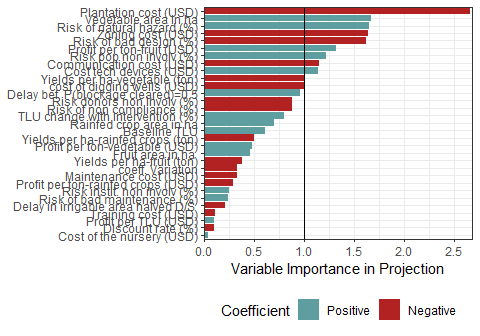#### Value of Information (VoI) analysis

We calculate Value of Information (VoI) analysis with the Expected Value of Perfect Information (EVPI). EVPI measures the expected opportunity loss that is incurred when the decision-maker does not have perfect information about a particular variable. EVPI is determined by examining the influence of that variable on the output value of a decision model.

We use the function data.frame() to transform the x and y outputs of the mcSimulation() function for EVPI calculation. We use the multi_EVPI() function to calculate the EVPI for multiple independent variables. For the first_out_var argument we choose ‘intervention_mngmt_audit_cost’ from the input table since this is the first variable after the NPV and cashflow model outputs, which we would like to exclude from the EVPI analysis.

#here we subset the outputs from the mcSimulation function (y) by selecting the correct variables
# this should be done by the user (be sure to run the multi_EVPI only on the variables that the user wants)
mcSimulation_table <- data.frame(mcSimulation_results$x, mcSimulation_results$y[1:3])

evpi <- multi_EVPI(mc = mcSimulation_table, first_out_var = "Interv_NPV")
#>  "Processing 3 output variables. This can take some time."
#>  "Output variable 1 (Interv_NPV) completed."
#>  "Output variable 2 (NO_Interv_NPV) completed."
#>  "Output variable 3 (NPV_decision_do) completed."

We use the function plot_evpi() on the results from multi_EVPI() to plot the Expected Value of Perfect Information (EVPI). Here we show the results with the standard settings. The length of the bars is equal to EVPI.


plot_evpi(evpi, decision_vars = "NPV_decision_do")
#> Warning: There are no variables with a positive EVPI. You probably do not need a
#> plot for that.

Finally, we provide a single function for a quick assessment. The compound_figure() function can be used to run the full decision assessment for a simple binary decision (‘do’ or ‘do not do’).

compound_figure(mcSimulation_object = mcSimulation_results, input_table = input_table, plsrResults = pls_result, EVPIresults = evpi, decision_var_name = "NPV_decision_do", cashflow_var_name = "Cashflow_decision_do", base_size = 7)
#> Warning: There are no variables with a positive EVPI. You probably do not need a
#> plot for that.